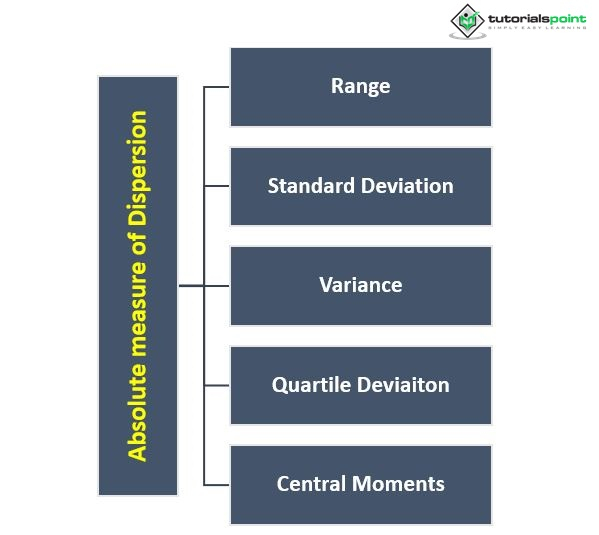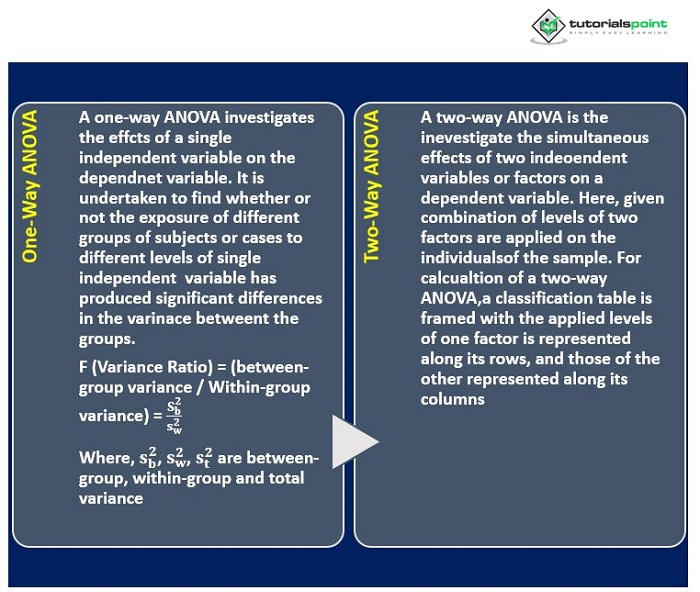# Application of Variance and Standard Deviation in Psychology

A variable's variability is the tendency of its scores to disperse from measurements of central tendency values such as a sample's mode, mean and median. The standard deviation and variance are worked out directly from the raw scores of a variable and are expressed in the same unit as that of those scores.

## Measures of Variability

Variability in statistics denotes the divergence of scores in a group or series from their mean scores. It refers to the spread of scores in the group about the mean. It is sometimes referred to as dispersion. For example, in a group of ten participants, each person differs from the others in terms of the marks he or she received on a mathematical examination. These fluctuations may be assessed using a measure of variability, which calculates the spread of distinct values for the average value or average score. The spread of values in a group is sometimes referred to as variability or dispersion. The high variability in the distribution indicates that the scores are dispersed and not homogenous. In statistics, variability refers to the variation of scores in a group or series from their mean scores. It truly refers to the group's score distribution about the mean. It is also known as dispersion. For example, in a group of ten participants, each person differs from the others in terms of the marks that he/she has received on a mathematical examination. These fluctuations may be assessed using a measure of variability, which measures the dispersion of distinct values for the average value or average score. Variability or dispersion also refers to the scatter of values within a group. The high variability in the distribution indicates that scores are dispersed and not homogenous## Variance

R.A. Fisher used the term variance to denote the square of the standard deviation in 1913. The idea of variance is crucial in advanced work when it is feasible to divide the total into numerous pieces, each of which is attributed to one of the reasons creating differences in their original series. The dispersion of a group of data points around their mean value is measured by variance. It is a mathematical expectation of the mean's average squared deviations. The variance (s2) or mean square (MS) is the arithmetic mean of individual scores' squared deviations from their averages. In other terms, it is the mean of the scores' squared deviations.

The variance and its closely related standard deviation are metrics showing how the distribution scores are spread out, and they are, in other words, variability measurements. The variance is calculated as the average squared departure from the mean of each integer. Many statistical applications and analyses need the calculation of a variance. It is an excellent absolute measure of variability and may be used to analyze variance (ANOVA) to determine the significance of variations in sample averages.

## Computation of Variance

For ungrouped scores, the sample variance is typically calculated as follows

$\mathrm{s^2={\frac{\sum(X-\bar{X})^2}{n-1}}}$

where, $\mathrm{\sum(X-\overline{X})^2}$ = sum of squared differences of scores from the sample mean, also known as the sum of squares

n = total number of frequencies

or,

$\mathrm{s^2={\frac{n\sum x^{2}-(\sum x)^2}{n(n-1)}}}$

where, $\mathrm{\sum X^2}$ = sum of squared scores

n = total number of frequencies

For a frequency distribution grouped into regular class intervals, variance is computed as the squared standard deviation by the following formula

$\mathrm{s^2=\frac{\sum f(x_c-\overline{x})^2}{n-1}}$

where, f = frequencies of the interval

Xc = midpoints of the intervals

The parametric or population variance is denoted by the symbol $\sigma^2$.

### Example

Compute the variance and SD of the following housefly wing lengths (mm)

3.5, 4.8, 4.3, 3.4, 5.1, 4.2, 3.8, 4.5, 3.6, 5.0, 3.4, 4.4, 5.3, 3.7, 4.0, 3.3

### Solution

After entering the scores (X) in Table 4.9, each X score is squared, and the squared scores (X2) are also entered in the table. X and X2 scores are totaled to give $\mathrm{\sum X}$ and $\mathrm{\sum X^2}$, respectively, which are used in working out the variance (s2) and the unbiased SD.

Serial No. Wing length (X) X2 Serial No. Wing length (X) X2
1 3.5 12.25 Total brought forward 37.2 156.64
2 4.8 23.04 10 5.0 25.00
3 4.3 18.49 11 3.4 11.56
4 3.4 11.56 12 4.4 19.36
5 5.1 26.01 13 5.3 28.09
6 4.2 17.64 14 3.7 13.69
7 3.8 14.44 15 4.0 16.00
8 4.5 20.25 16 3.3 10.89
9 3.6 12.96 9 66.3 281.23
Total carried forward 37.2 156.64 Total ($\mathrm{\sum X}$) ($\mathrm{\sum X^2}$)

$\mathrm{s^2=\frac{n \sum x^2 − (\sum x)^2}{n(n-1)}}$

$\frac{(16 \times 281.23 − 66.32)}{16(16 − 1)}$

$\mathrm{= 0.433mm^2}$

$\mathrm{s = \sqrt{s^2} = \sqrt{0.433} = 0.658 \: mm}$

## Analysis of Variance (ANOVA)

An experiment is designed to investigate the impact of one or more independent factors on one or more dependent variables. ANOVA is used to determine whether or not the sample's exposure to the independent variable increased the variance of the dependent variable significantly above the variation attributable to random causes. The main goal is to calculate the likelihood that the means of three or more groups of scores differ due to sampling error.

## Types of ANOVA

Major types are## Standard Deviation (SD)

Carl Pearson proposed the standard deviation, indicated by s or $\sigma$, to measure dispersion in 1893. The arithmetic mean of the squares of the departure from the arithmetic means is defined as the positive square root of that number. Karl Pearson used the phrase "standard deviation" for the first time in writing in 1894. The population standard deviation is indicated by "(Greek letter sigma), while the sample standard deviation is denoted by '$\sigma$.' SD is useful because, unlike variance, it is represented in the same unit as the data. This is the most often utilized method of variation. The standard deviation represents the average of all the scores around the mean. It is the positive square root of the mean of all the squared deviations from the mean. It is the square root of the positive variance.

The standard deviation indicates the variance from the mean, and SD is determined solely based on the mean. A low standard deviation indicates that the data is near the mean, and a high standard deviation shows that the data is spread over a wide range of values. The standard deviation can be used to assess uncertainty. The standard deviation offers the information if you wish to test the theory or evaluate whether measurements agree with a theoretical prediction. If the mean and standard deviation difference is particularly big, the theory being tested should be updated.

The mean with the lowest standard deviation is more dependable than the highest standard deviation, and a lower SD indicates that the data is more homogeneous. The standard deviation value is calculated for each observation in data collection. SD is utilized in the subsequent statistical analysis since it is the only measure of dispersion that can be treated algebraically.

Standard deviation is used when

• The statistic having the greatest stability is sought, and we need the most reliable and accurate measure of variability.

• Extreme deviation should exercise a proportionally greater effect upon the variability, and when the distribution is normal or near normal.

## Calculation

$\mathrm{s=\sqrt{\frac{\overline{\sum(X-\overline{X})^2}}{n}}={\sqrt{\frac{\sum X^2}{n}}}}$

where, X= individual scores

$\mathrm{\overline{X}}$ = sample mean

$\mathrm{(X − \overline{X})}$ or x = deviation of a score from $\mathrm{\overline{X}}$

• It is rigidly defined and based on all the observations of the distribution.

• It is a more stable or accurate estimate of the population parameter than other measures of variation.

• The SD is affected the least by the sampling fluctuation of all the measures of dispersion.

• It is possible to determine the combined SDs of two or more groups.

• It is prominently used in further statistical work. For example: in computing skewness and kurtosis, correlation and regression, as well as a test of significance

• The standard deviation is a keystone in sampling and provides a unit of measurement for the normal deviation.

## Conclusion

Measures such as variance or standard deviation cannot be used in comparing the dispersions or variabilities of the scores of more than one variable expressed in different units. Moreover, these absolute measures are not suitable for comparing the variabilities of two sets of scores expressed in the same unit but having widely divergent and central values.

Updated on: 30-Dec-2022

624 Views